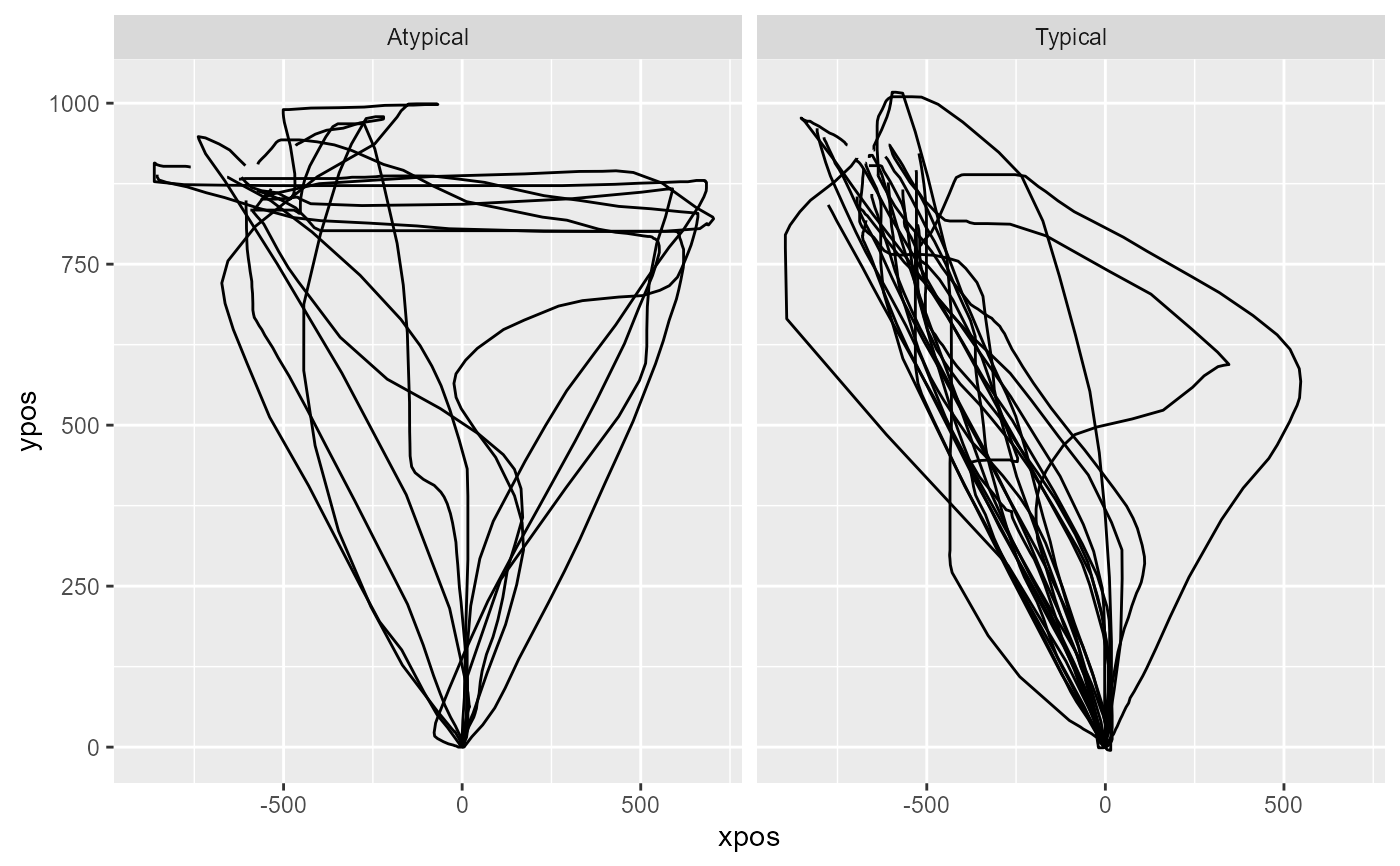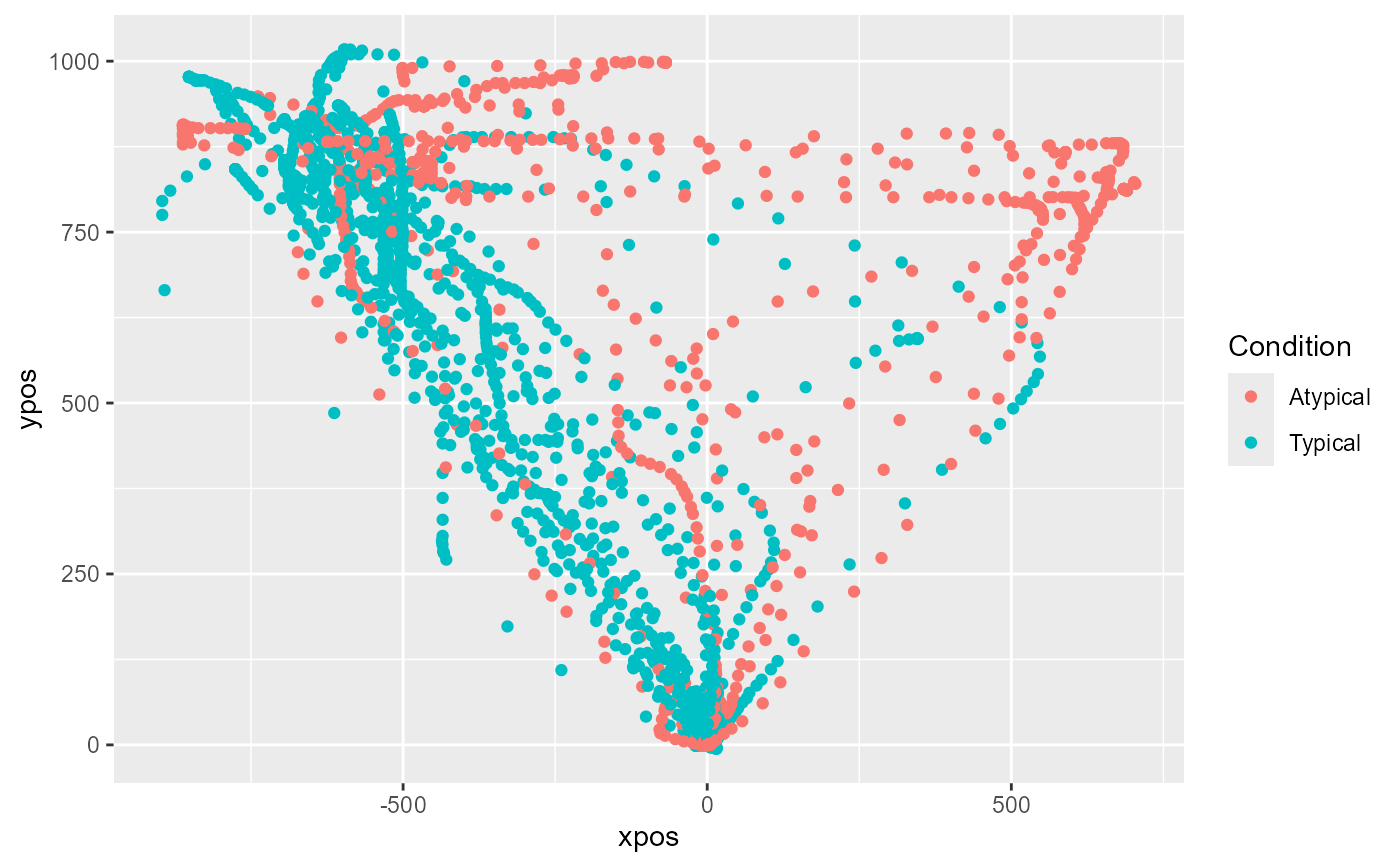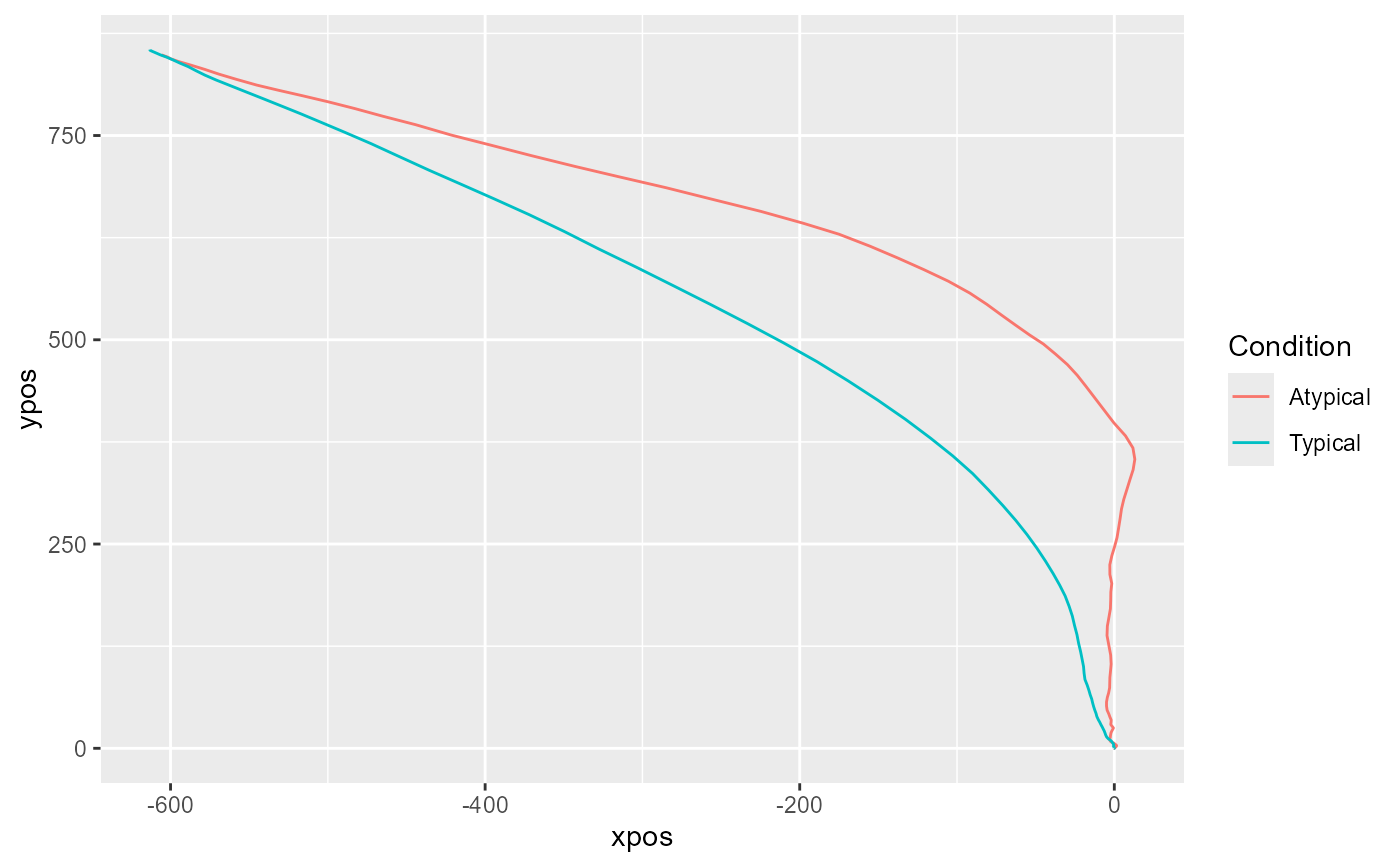mt_plot can be used for plotting a number of individual trajectories. mt_plot_aggregate can be used for plotting aggregated trajectories. The color and linetype can be varied depending on a set of condition variables using the color and linetype arguments. If the x and y arguments are varied, this function can also be used for plotting velocity and acceleration profiles.

mt_plot(data, use = "trajectories", use2 = "data", x = "xpos",
y = "ypos", color = NULL, linetype = NULL, alpha = NA,
size = 0.5, facet_row = NULL, facet_col = NULL, points = FALSE,
only_ggplot = FALSE, mt_id = "mt_id", ...)

mt_plot_aggregate(data, use = "trajectories", use2 = "data",
x = "xpos", y = "ypos", color = NULL, linetype = NULL,
alpha = NA, size = 0.5, facet_row = NULL, facet_col = NULL,
points = FALSE, only_ggplot = FALSE, subject_id = NULL, ...)

## Arguments

data a mousetrap data object created using one of the mt_import functions (see mt_example for details). Alternatively, a trajectory array can be provided directly (in this case use will be ignored). a character string specifying which trajectories should be plotted. The corresponding trajectories are selected from data using data[[use]]. Usually, this value corresponds to either "trajectories", "tn_trajectories" or "av_trajectories", depending on whether the raw, time-normalized or averaged trajectories should be plotted. a character string specifying where the data that contain the variables used for determining the color and linetype can be found (in case these arguments are specified). Defaults to "data" as data[["data"]] usually contains all non mouse-tracking trial data. a character string specifying which dimension in the trajectory array should be displayed on the x-axis (defaults to xpos). a character string specifying which dimension in the trajectory array should be displayed on the y-axis (defaults to ypos). an optional character string specifying which variable in data[[use2]] should be used for coloring the trajectories. an optional character string specifying which variable in data[[use2]] should be used for varying the linetype of the trajectories. an optional numeric value between 0 and 1 that can be used to make the plotted lines (and points) semitransparent. an optional numeric value that can be used to vary the width of the plotted trajectory lines. an optional character string specifying a variable in data[[use2]] that should be used for (row-wise) faceting. an optional character string specifying a variable in data[[use2]] that should be used for (column-wise) faceting. logical. If TRUE, points will be added to the plot using geom_point. logical. If TRUE, only the ggplot object without geoms is returned. If FALSE (the default), the trajectories are plotted using geom_path. a character string specifying the internal label used for the trial identifier (passed on to the group aesthetic). Only relevant for mt_plot. additional arguments passed on to mt_reshape (such as subset). a character string specifying which column contains the subject identifier. Only relevant for mt_plot_aggregate. If specified, aggregation will be performed within subjects first. Note that aggregation will be performed separately for each level, including all subjects for whom data are available.

## Details

mt_plot internally uses mt_reshape for reshaping trajectories into a long format. Next, it creates a ggplot object using the ggplot function of the ggplot2 package. The aes mappings are taken from the function arguments for x, y etc.; in addition, the group mapping is set to the internal trial identifier (by default called "mt_id").

If only_ggplot==FALSE, the trajectories are plotted using the geom_path function of the ggplot2 package. If only_ggplot==TRUE, the ggplot object is returned without layers, which can be used to further customize the plot, e.g., to specify a custom size for the lines or to create semitransparent lines by specifying an alpha level < 1 (see Examples).

mt_plot_aggregate works similarly, but uses mt_aggregate for reshaping and aggregating trajectories prior to plotting.

Please note that this function is intended as a quick and easy solution for visualizing mouse trajectories. For additional flexibility, we recommend that mt_reshape or mt_aggregate be used in conjunction with ggplot to create custom visualizations.

## Functions

• mt_plot: Plot individual trajectory data

• mt_plot_aggregate: Plot aggregated trajectory data

mt_plot_add_rect for adding rectangles representing the response buttons to the plot.

mt_plot_riverbed for plotting the relative frequency of a selected variable across time.

mt_plot_per_trajectory for individually plotting all trajectories as individual pdf files.

## Examples

# Load ggplot2
library(ggplot2)

## Plot individual example trajectories

# Time-normalize trajectories
mt_example <- mt_time_normalize(mt_example)

# Plot all time-normalized trajectories
# varying the color depending on the condition
mt_plot(mt_example, use="tn_trajectories",
color="Condition")# ... with custom colors
mt_plot(mt_example, use="tn_trajectories",
color="Condition") +
ggplot2::scale_color_brewer(type="qual")# Create separate plots per Condition
mt_plot(mt_example, use="tn_trajectories",
facet_col="Condition")# Plot velocity profiles based on the averaged trajectories
# varying the color depending on the condition
mt_example <- mt_derivatives(mt_example)
mt_example <- mt_average(mt_example, interval_size = 100)
mt_plot(mt_example, use="av_trajectories",
x="timestamps", y="vel", color="Condition")## Plot aggregate trajectories for KH2017 data

# Time-normalize trajectories
KH2017 <- mt_time_normalize(KH2017)

# Plot aggregated time-normalized trajectories per condition
mt_plot_aggregate(KH2017, use="tn_trajectories",
color="Condition")# ... first aggregating trajectories within subjects
mt_plot_aggregate(KH2017, use="tn_trajectories",
color="Condition", subject_id="subject_nr")# ... adding points for each position to the plot
mt_plot_aggregate(KH2017, use="tn_trajectories",
color="Condition", points=TRUE)# NOT RUN {
# Create customized aggregate trajectory plot
# by using only_ggplot option to return a ggplot object without geoms
# and by adding a geom to it with a custom line width
mt_plot_aggregate(KH2017, use="tn_trajectories",
color="Condition", only_ggplot=TRUE) +
geom_path(size=1.5)

# Create customized plot of individual trajectories
# by using only_ggplot option to return a ggplot object without geoms
# and by adding a geom to it with semitransparent lines
# (by specifying alpha < 1)
mt_plot(KH2017, use="tn_trajectories", only_ggplot=TRUE) +
geom_path(alpha=0.2)
# }